# Bihar Board 12th Maths Objective Answers Chapter 5 Continuity and Differentiability

Bihar Board 12th Maths Objective Questions and Answers

## Bihar Board 12th Maths Objective Answers Chapter 5 Continuity and Differentiability

Question 1.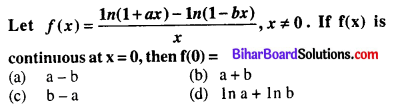(b) ln a + ln b

Question 2.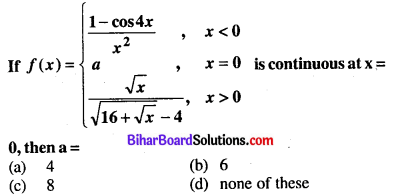(c) 8

Question 3.
The number of discontinuous functions y(x) on [-2, 2] satisfying x2 + y2 = 4 is
(a) 0
(b) 1
(c) 2
(d) >2
(a) 0

Question 4.(c) $$-\frac{1}{2}$$

Question 5.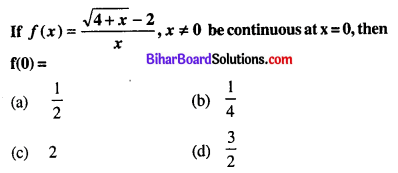(b) $$\frac{1}{4}$$

Question 6.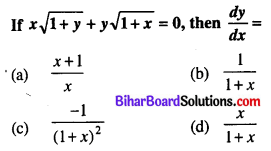(c) $$\frac{-1}{(1+x)^{2}}$$

Question 7.
If y = (1 + x)(1 + x2)(1 + x4)…..(1 + x2n), then the value of $$\frac{d y}{d x}$$ at x = 0 is
(a) 0
(b) -1
(c) 1
(d) None of these
(c) 1

Question 8.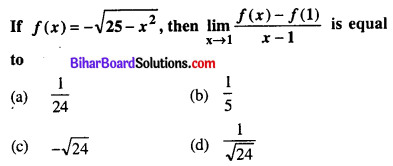(d) $$\frac{1}{\sqrt{24}}$$

Question 9.
If y = ax2 + b, then $$\frac{d y}{d x}$$ at x = 2 is equal to
(a) 4a
(b) 3a
(c) 2a
(d) None of these
(a) 4a

Question 10.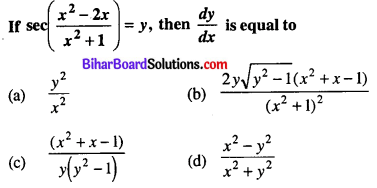(b) $$\frac{2 y \sqrt{y^{2}-1}\left(x^{2}+x-1\right)}{\left(x^{2}+1\right)^{2}}$$

Question 11.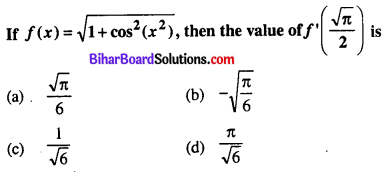(b) $$-\sqrt{\frac{\pi}{6}}$$

Question 12.(a) $$\frac{\sqrt{(x+y)}-\sqrt{y-x}}{\sqrt{y-x}+\sqrt{x+y}}$$

Question 13.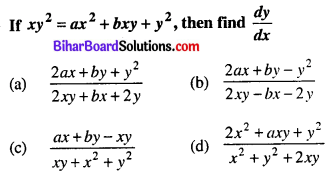(b) $$\frac{2 a x+b y-y^{2}}{2 x y-b x-2 y}$$

Question 14.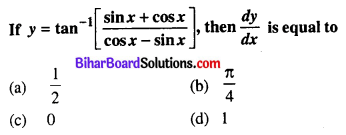(d) 1

Question 15.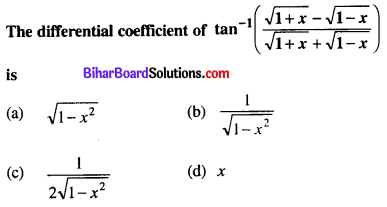(c) $$\frac{1}{2 \sqrt{1-x^{2}}}$$

Question 16.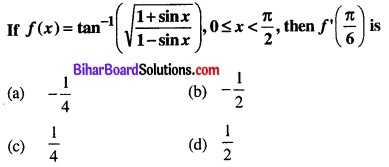(d) $$\frac{1}{2}$$

Question 17.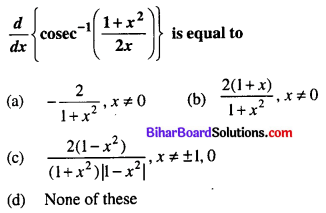(c) $$\frac{2\left(1-x^{2}\right)}{\left(1+x^{2}\right)\left|1-x^{2}\right|}, x \neq\pm 1,0$$

Question 18.(b) 0

Question 19.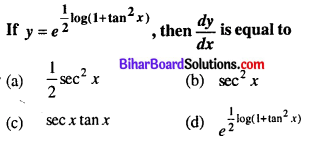(c) sec x tan x

Question 20.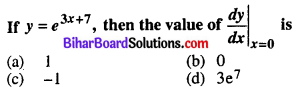(d) 3e7

Question 21.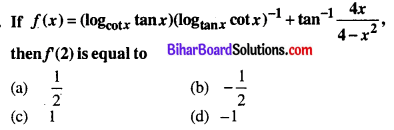(a) $$\frac{1}{2}$$

Question 22.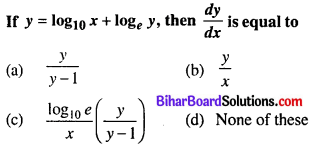(c) $$\frac{\log _{10} e}{x}\left(\frac{y}{y-1}\right)$$

Question 23.(d) None of these

Question 24.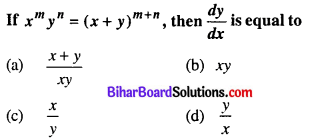(d) $$$\frac{y}{x}$$$

Question 25.
If Rolle’s theorem holds for the function f(x) = x3 + bx2 + ax + 5 on [1, 3] with c = (2 + $$\frac{1}{\sqrt{3}}$$), find the value of a and b.
(a) a = 11, b = -6
(b) a = 10, b = 6
(c) a = -11, b = 6
(d) a = 11, b = 6
(a) a = 11, b = -6

Question 26.
If y = (tan x)sin x, then $$\frac{d y}{d x}$$ is equal to
(a) sec x + cos x
(b) sec x + log tan x
(c) (tan x)sin x
(d) None of these
(d) None of these

Question 27.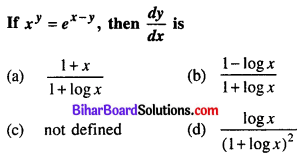(d) $$\frac{\log x}{(1+\log x)^{2}}$$

Question 28.
The derivative of y = (1 – x)(2 – x) ….. (n – x) at x = 1 is equal to
(a) 0
(b) (-1)(n – 1)!
(c) n! – 1
(d) (-1)n-1(n – 1)!
(b) (-1)(n – 1)!

Question 29.
If xy . yx = 16, then the value of $$\frac{d y}{d x}$$ at (2, 2) is
(a) -1
(b) 0
(c) 1
(d) none of these
(a) -1

Question 30.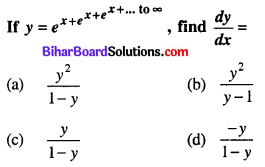(c) $$\frac{y}{1-y}$$

Question 31.
The derivative of f(tan x) w.r.t. g(sec x) at x = $$\frac{\pi}{4}$$, where f'(1) = 2 and g'(√2) = 4, is
(a) $$\frac{1}{\sqrt{2}}$$
(b) √2
(c) 1
(d) 0
(a) $$\frac{1}{\sqrt{2}}$$

Question 32.(c) $$\frac{2}{3}$$

Question 33.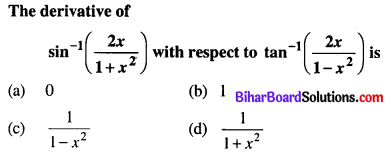(b) 1

Question 34.(c) $$\frac{5}{16 t^{6}}$$

Question 35.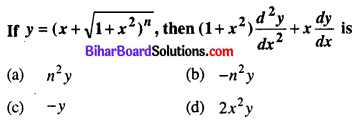(a) n2y

Question 36.(d) $$-\frac{b}{a^{2}} \sec ^{3} \theta$$

Question 37.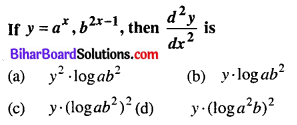(c) y. (log ab2)2

Question 38.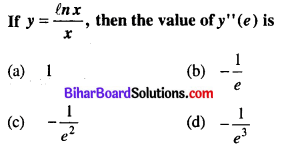(d) $$-\frac{1}{e^{2}}$$

Question 39.(a) $$\frac{\sec ^{3} \theta}{a \theta}$$

Question 40.(d) 0

Question 41.
If x2 + y2 = 1, then
(a) yy” – (2y’)2 + 1 = 0
(b) yy” + (y’)2 + 1 = 0
(c) yy” – (y’)2 – 1 = 0
(d) yy” + (2y’)2 + 1 = 0
(b) yy” + (y’)2 + 1 = 0

Question 42.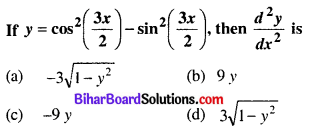(c) -9y

Question 43.
The value of c in Rolle’s theorem for the function, f(x) = sin 2x in [0, $$\frac{\pi}{2}$$] is
(a) $$\frac{\pi}{2}$$
(b) $$\frac{\pi}{4}$$
(c) $$\frac{\pi}{3}$$
(d) $$\frac{\pi}{6}$$
(b) $$\frac{\pi}{4}$$

Question 44.
The value of c in Rolle’s Theorem for the function f(x) = ex sin x, x ∈ [0, π] is
(a) $$\frac{\pi}{6}$$
(b) $$\frac{\pi}{4}$$
(c) $$\frac{\pi}{2}$$
(d) $$\frac{3 \pi}{4}$$
(d) $$\frac{3 \pi}{4}$$

Question 45.
A value of c for which the Mean value theorem holds for the function f(x) = logex on the interval [1, 3] is
(a) 2log3e
(b) $$\frac{1}{2} \log _{e} 3$$
(c) log3e
(d) loge3
(a) 2log3e

Question 46.
The value of c in mean value theorem for the function f(x) = (x – 3)(x – 6)(x – 9) in [3, 5] is
(a) 6 ± √(13/3)
(b) 6 + √(13/3)
(c) 6 – √(13/3)
(d) None of these
(a) $$\frac{3}{2}$$
(b) $$\frac{2}{3}$$
(c) $$\frac{1}{2}$$
(d) $$\frac{5}{2}$$
(a) $$\frac{3}{2}$$